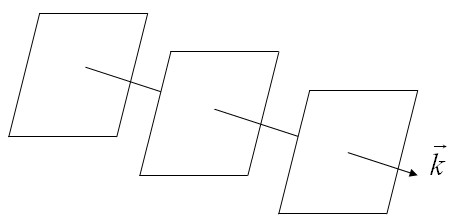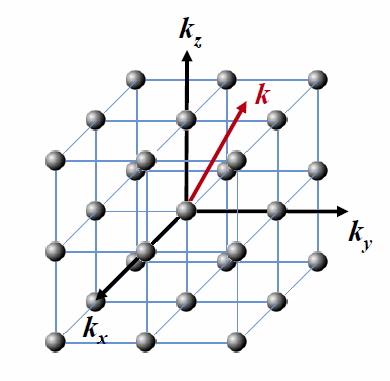Menu Outline Exercise Questions Appendices Lectures Student Projects Books Sections Introduction Atoms Molecules Crystal Structure Crystal Diffraction Crystal Binding Photons Phonons Electrons Band Model Crystal Physics Semiconductors

PHY.K02UF Molecular and Solid State Physics

## Plane waves and reciprocal space

The complex exponentials such as those that are used for a Fourier series are often called plane waves. A plane wave has the form,

$$e^{i\vec{k}\cdot\vec{r}} = \cos \left(\vec{k}\cdot\vec{r}\right) + i\sin \left(\vec{k}\cdot\vec{r}\right).$$

Here $\vec{k} = k_x\,\hat{x}+k_y\,\hat{y}+k_z\,\hat{z}$ is a vector called a wave vector. Consider the value of the plane wave at a particular point $\vec{r}$. In general, the value at this point is some complex number. Now move away from that point in a direction perpendicular to $\vec{k}$ to $\vec{r}+\vec{r}_{\perp}$. Every point on the plane that passes through $\vec{r}$ and is perpendicular to $\vec{k}$ has the same value, $e^{i\vec{k}\cdot\left(\vec{r} +\vec{r}_{\perp}\right)} = e^{i\vec{k}\cdot\vec{r}}$.If you move in a direction parallel to $\vec{k}$, the function oscillates with a wavelength $\lambda = 2\pi/|\vec{k}|$. The real parts of some plane waves are plotted below. You just have to imagine the imaginary parts.

 $\vec{k}=6\,\hat{x}$ $\vec{k}=6\,\hat{y}$ $\vec{k}=4\,\hat{x}+4\,\hat{y}$ $\vec{k}=3\,\hat{x}-5\,\hat{y}$

### Reciprocal space

Reciprocal space is a space of plane waves. Every point in a reciprcal space spanned by the vectors $\vec{k}=k_x\hat{k}_x+k_y\hat{k}_y+k_y\hat{k}_y$ corresponds to a plane wave. The direction of the $k$-vector indicates the direction the plane wave is moving and $2\pi/|\vec{k}|$ is the wavelength of the plane wave. A distance $|\vec{k}|$ measured in reciprocal space is measured in unit of 1/meters and this is why the space is reciprocal. A reciprocal lattice is a periodic arrangement of points in reciprocal space.The red vector is a $k$-vector in reciprocal space. The black points represent a reciprocal lattice.

We have found that a discrete sum of plane waves can be used to describe a periodic function,

$$f\left(\vec{r}\right)= \sum\limits_{\vec{G}} f_{\vec{G}}e^{i\vec{G}\cdot\vec{r}}.$$

Here the reciprocal lattice points $\vec{G}$ are a lattice of points in reciprocal space. Now consider functions that can be expressed as a continuous sum of plane waves,

$$f\left(\vec{r}\right)= \int F\left(\vec{k}\right)e^{i\vec{k}\cdot\vec{r}}d\vec{k} .$$

The integral in this last expression extends over all values of $\vec{k}$ in reciprocal space. The function $F\left(\vec{k}\right)$ is a weighting function that tells us how much of plane wave $\vec{k}$ should be included in the sum. The quantity $F\left(\vec{k}\right)$ called the Fourier transform of $f\left(\vec{r}\right)$ and is a function in $k$-space. For physically relevant quantities, $F\left(\vec{k}\right)$ typically goes to zero for large values of $|\vec{k}|$ so that the integral is well defined.

All of the information needed to specify $f\left(\vec{r}\right)$ is contained in $F\left(\vec{k}\right)$. The converse is also true. The Fourier transform $F\left(\vec{k}\right)$ of $f\left(\vec{r}\right)$ can be calculated by,

$$F\left(\vec{k}\right)= \frac{1}{\left( 2\pi \right)^d} \int f\left(\vec{r}\right)e^{-i\vec{k}\cdot\vec{r}}d\vec{r} .$$

Here $d$ is the number of dimensions the function $f\left(\vec{r}\right)$ is defined in.